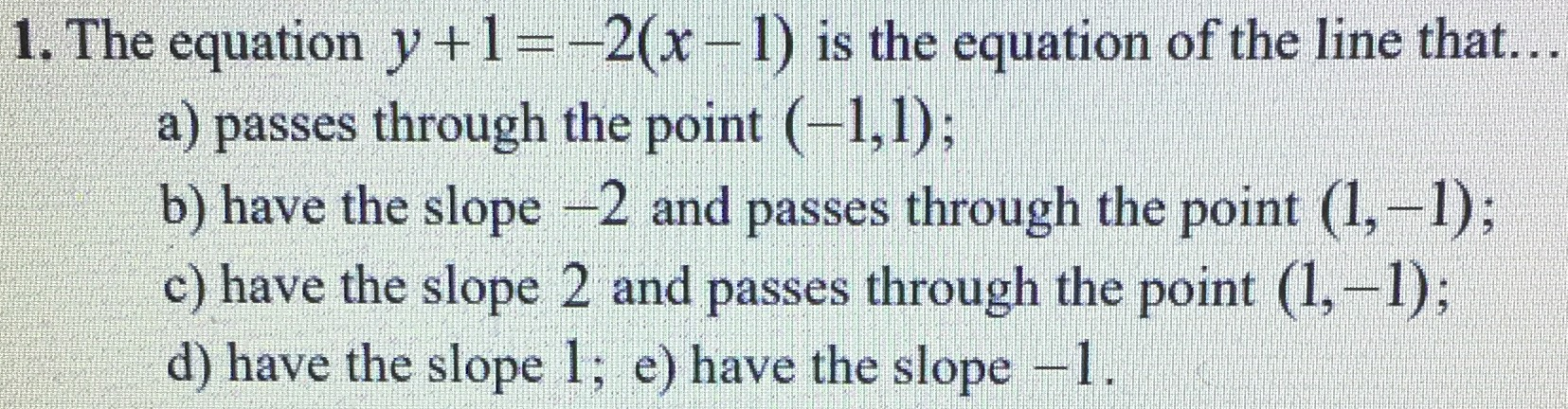### ¿Todavía tienes preguntas de matemáticas?

Pregunte a nuestros tutores expertos
Algebra
Pregunta1. The equation $$y + 1 = - 2 ( x - 1 )$$ is the equation of the line that... a) passes through the point $$( - 1,1 )$$ ; b) have the slope $$- 2$$ and passes through the point $$( 1 , - 1 )$$ ; c) have the slope $$2$$ and passes through the point $$( 1 , - 1 )$$ ; d) have the slope $$1$$ ; e) have the slope $$- 1$$ .